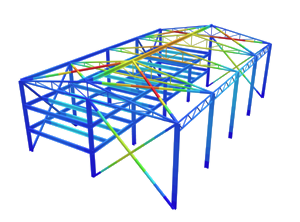SkyCiv Documentation

Your guide to SkyCiv software - tutorials, how-to guides and technical articles

1. Home
4. NBCC 2015 Snow Load Calculation

# NBCC 2015 Snow Load Calculation

## Using the SkyCiv Load Generator in NBCC 2015 Snow Load Calculations

To calculate the snow load pressures for a structure using SkyCiv Load Generator, the process is to define first the code reference. From there, the workflow is to define the parameters in Project Tab, Site Tab, and Building Tab, respectively. However, only users with a Professional Account or who purchased the standalone Load Generator module will be able to use this calculation. You can purchase the standalone module thru this link.

## Site Data

Using NBCC 2015, you need to select Recurrence Interval, Limit State, and Importance Category parameters first before putting an address the Project Address input. The module will search for the location based on Table C-2 of Appendix C (NBCC 2015).

You can manually put the ground snow load and the associated rain load whenever needed. Moreover, Area Classification should be defined in order to calculate for Cw.

### Structure Data

The structure data and the wind and snow parameters are separated into different accordions. In order to calculate snow loads, the snow load checkbox should be checked.

#### Sloped Roof Condition

This parameter is used in calculating Slope Factor, Cs. The options for this parameter are: “Unobstructed slippery” and “Other Cases”

#### Consider Unbalanced/Drifted Snow Load Case?

This parameter is a “Yes” or “No” to calculate for Unbalanced Snow Load Case. Upon selecting “No,” the balanced snow load case shall only be calculated. On the other hand, upon selecting “Yes,” the unbalanced snow load case table will show. There are five options for NBCC 2015:

Multi-level Roofs – Case 1

This case is used in calculating snow load drift on lower roofs where the wind source is coming from the upper roof as shown in figure below based on Figure 4.1.6.5-A and 4.1.6.5-B Case I of NBCC 2015.

Multi-level Roofs – Case 2

Similar to the previous case, this is used in calculating snow load drift on lower roofs where the wind source is coming from the lower roof as shown in figure below based on Figure 4.1.6.5-A and 4.1.6.5-B Case II of NBCC 2015.

Multi-level Roofs – Case 3

This case is used in calculating snow load drift on lower roofs where the wind source is coming from the lower roof on a diagonal direction as shown in figure below based on Figure 4.1.6.5-A and 4.1.6.5-B Case III of NBCC 2015.

This case is used in calculating snow drifts on areas adjacent to roof projections such as parapets, chimneys, etc.

Gable Roofs

This case is used to calculate the snow load drift on symmetric gable roof.

### Results

The snow load results will show the parameters in calculating the balanced snow load as shown below:

If Unbalanced/Drifted Snow Load is selected to be considered, the results are tabulated on a table depending on the cases defined (sample below):